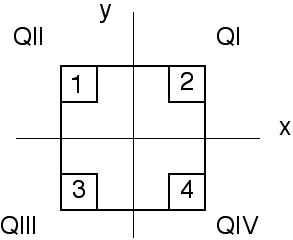The figure shows a uniform square plate from which four identical squares at the corners will be removed.

(a) Where is the center of mass of the plate originally?
(b) In which quadrant is the center of mass after the removal of square 1?
(c) Where is it after removal of squares 1 and 2 ?
(d) Where is it after removal of square 1 and 3 ?
(e) Where is it after removal of squares 1,2 and 3?
(f) Where is it after removal of all four squares?